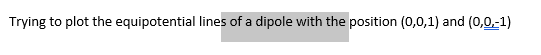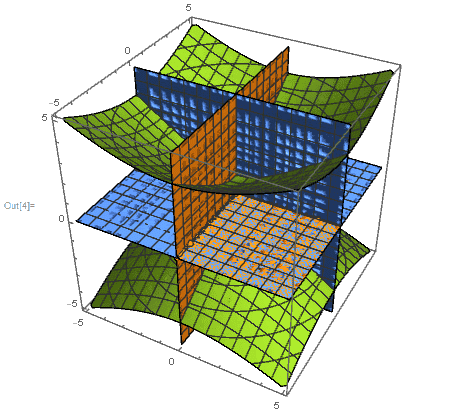# Mathematica plotting

• Ashley1nOnly

## Homework Statement## Homework Equations

E= kq/r^2 * r-hat

where q= +/-(1.6*10^-19)

## The Attempt at a Solution

x=

(1.6*10^-19)*x/(x^2+y^2+(z+1)^2)^(3/2) - (1.6*10^-19)*x/(x^2+y^2+(z-1)^2)^(3/2)

y=

(1.6*10^-19)*y/(x^2+y^2+(z+1)^2)^(3/2) - (1.6*10^-19)*y/(x^2+y^2+(z-1)^2)^(3/2)

z=

(1.6*10^-19)*(z+1)/(x^2+y^2+(z+1)^2)^(3/2) - (1.6*10^-19)*(z-1)/(x^2+y^2+(z-1)^2)^(3/2)

ContourPlot3D[{x,y,z},{x,-5,5},{y,-5,5},{z,-5,5}]

This should work but it is giving me a recursion limit error. I know that the math (well at least I think) is correct.

Any suggestions on how to fix the error?

## Homework Statement

View attachment 230602

## Homework Equations

E= kq/r^2 * r-hat

where q= +/-(1.6*10^-19)

## The Attempt at a Solution

x=

(1.6*10^-19)*x/(x^2+y^2+(z+1)^2)^(3/2) - (1.6*10^-19)*x/(x^2+y^2+(z-1)^2)^(3/2)

y=

(1.6*10^-19)*y/(x^2+y^2+(z+1)^2)^(3/2) - (1.6*10^-19)*y/(x^2+y^2+(z-1)^2)^(3/2)

z=

(1.6*10^-19)*(z+1)/(x^2+y^2+(z+1)^2)^(3/2) - (1.6*10^-19)*(z-1)/(x^2+y^2+(z-1)^2)^(3/2)

ContourPlot3D[{x,y,z},{x,-5,5},{y,-5,5},{z,-5,5}]

This should work but it is giving me a recursion limit error. I know that the math (well at least I think) is correct.

Any suggestions on how to fix the error?

I haven't checked your physics; the rest of this reply is solely involving the recursion limit error.

You are using your x, y, and z variables for different things in different places. Try changing the names of your variables appropriately.

For example, right now you have
x = a function of x (and also y and z).

Try changing that to

x1 = a function of x (and also y and z)

or some other variable name that makes more sense.

Thanks, that worked. It gave me an answer with the expected lines but it does look funny.